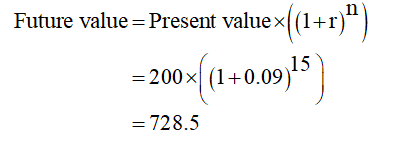# For the cash flows shown, calculate the future worth in year 15 using i = 9% per year.Year0123456Cash flow, \$200200200200300300300 The future worth is calculated to be \$

Question
18 views

For the cash flows shown, calculate the future worth in year 15 using i = 9% per year.

 Year 0 1 2 3 4 5 6 Cash flow, \$ 200 200 200 200 300 300 300

The future worth is calculated to be \$

check_circle

star
star
star
star
star
1 Rating
Step 1

To get the future worth amount, we have to add each year’s future worth beaded on the cash flow of respective years.

Step 2

We can calculate the future worth amount using the following equation.Step 3

Suppose, for year 0, the cash flow is \$200 and it get complete 15 years to grow at 9% per year, we can calculate the future worth amount for the particular year as follows....

### Want to see the full answer?

See Solution

#### Want to see this answer and more?

Solutions are written by subject experts who are available 24/7. Questions are typically answered within 1 hour.*

See Solution
*Response times may vary by subject and question.
Tagged in